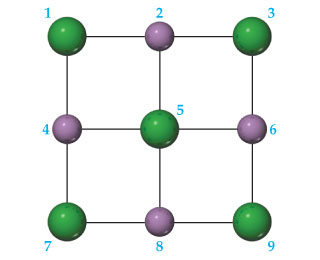# Problem: A portion of a two-dimensional "slab" of NaCl(s) is shown here in which the ions are numbered.Consider ion 5. How many repulsive interactions are shown for it?

🤓 Based on our data, we think this question is relevant for Professor Ratliff's class at USF.

###### FREE Expert Solution

We have to determine how many repulsive interactions are shown for ion 5 in the given diagram.

We will start by the fact that opposite charges attract each other, and same charges repel each other.

In the given diagram, the sodium ions (Na+) are shown by purple spheres and the chloride ions (Cl-) are shown by green spheres.###### Problem Details
A portion of a two-dimensional "slab" of NaCl(s) is shown here in which the ions are numbered.Consider ion 5. How many repulsive interactions are shown for it?